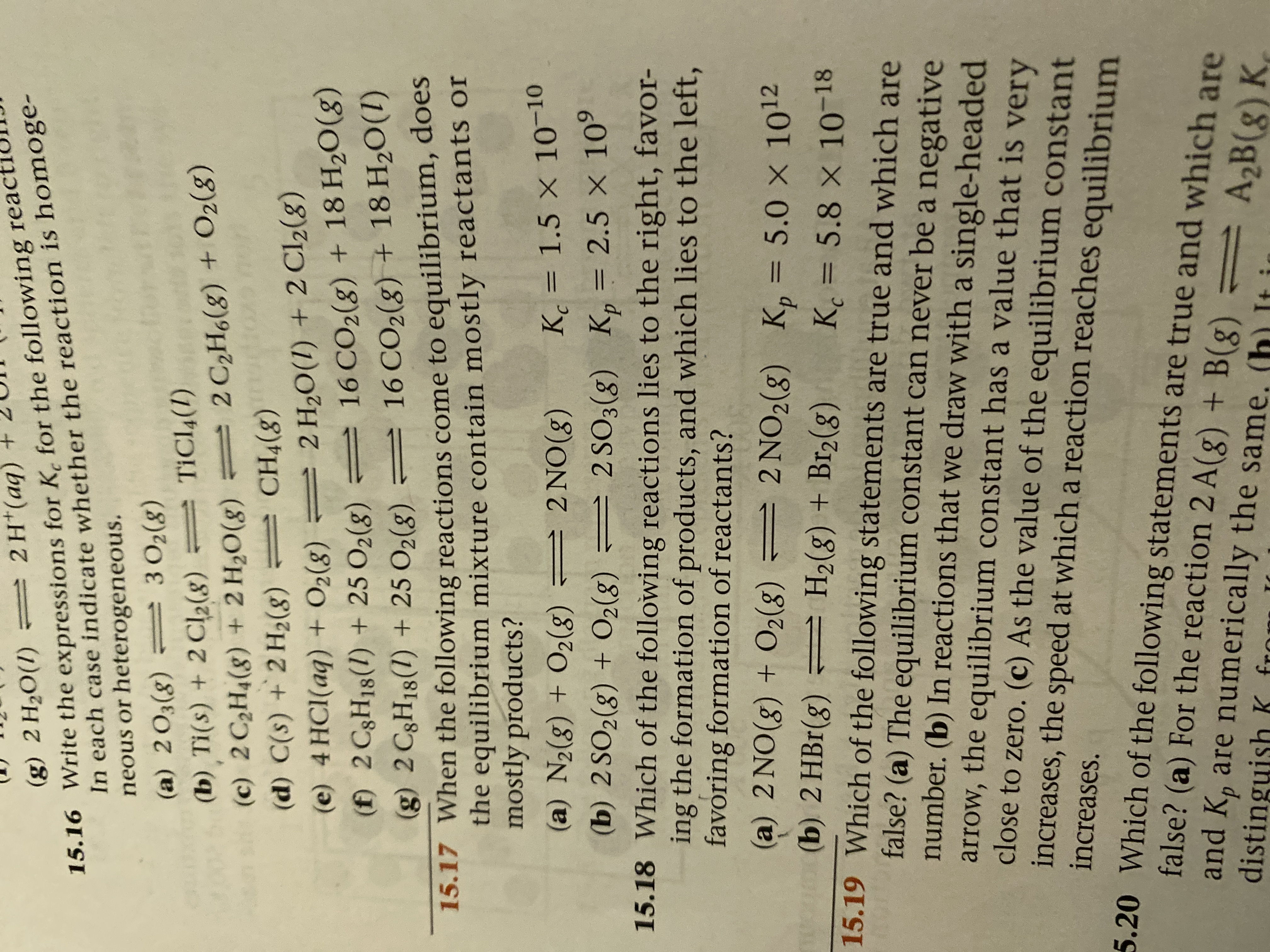# = 2H*(aq)In each case indicate whether the reaction is homoge-neous or heterogeneous.(g) 2 H2O(1)15.16 Write the expressions for K, for the following react3 O2(8)= TICL4(1)(a) 2O3(g) = 302(g)(b) Ti(s) + 2 Cl2(8)(c) 2 C2H4(8) + 2 H20(8)(d) C(s) +2 H2(8) CH4(8)(e) 4 HCI(aq) + O2(g) = 2 H2O(1) + 2 Cl2(8)(f) 2 C3H18(1) + 25 O2(8)(g) 2 C3H18(1) + 25 O2(g) = 16 CO2(g) + 18 H2O(1)2 C2H6(g) + O2(8)=16 CO2(8) + 18 H2O(g)=15.17 When the following reactions come to equilibrium, doesthe equilibrium mixture contain mostly reactants ormostly products?Kc = 1.5 × 10-10(a) N2(g) + O2(8) = 2 NO(8)%3D(b) 2 SO2(g) + O2(8) = 2 SO3(8) K, = 2.5 × 10°15.18 Which of the following reactions lies to the right, favor-ing the formation of products, and which lies to the left,favoring formation of reactants?%3D(a) 2 NO(8) + O2(8) = 2 NO2(g) K,= 5.0 × 1012(b) 2 HBr(8)%3Dmo(= H2(g) + Br2(g)15.19 Which of the following statements are true and which arefalse? (a) The equilibrium constant can never be a negativenumber. (b) In reactions that we draw with a single-headedarrow, the equilibrium constant has a value that is veryclose to zero. (c) As the value of the equilibrium constantincreases, the speed at which a reaction reaches equilibriumK =5.8x 10-18%3Dincreases.5.20 Which of the following statements are true and which arefalse? (a) For the reaction 2 A(g) + B(g) = A,B(g) Kand K, are numerically the same. (h) It idistinguish K fro

Question
1 views

see photo. Problem # 15.18help_outlineImage Transcriptionclose= 2H*(aq) In each case indicate whether the reaction is homoge- neous or heterogeneous. (g) 2 H2O(1) 15.16 Write the expressions for K, for the following react 3 O2(8) = TICL4(1) (a) 2O3(g) = 302(g) (b) Ti(s) + 2 Cl2(8) (c) 2 C2H4(8) + 2 H20(8) (d) C(s) +2 H2(8) CH4(8) (e) 4 HCI(aq) + O2(g) = 2 H2O(1) + 2 Cl2(8) (f) 2 C3H18(1) + 25 O2(8) (g) 2 C3H18(1) + 25 O2(g) = 16 CO2(g) + 18 H2O(1) 2 C2H6(g) + O2(8) = 16 CO2(8) + 18 H2O(g) = 15.17 When the following reactions come to equilibrium, does the equilibrium mixture contain mostly reactants or mostly products? Kc = 1.5 × 10-10 (a) N2(g) + O2(8) = 2 NO(8) %3D (b) 2 SO2(g) + O2(8) = 2 SO3(8) K, = 2.5 × 10° 15.18 Which of the following reactions lies to the right, favor- ing the formation of products, and which lies to the left, favoring formation of reactants? %3D (a) 2 NO(8) + O2(8) = 2 NO2(g) K,= 5.0 × 1012 (b) 2 HBr(8) %3D mo( = H2(g) + Br2(g) 15.19 Which of the following statements are true and which are false? (a) The equilibrium constant can never be a negative number. (b) In reactions that we draw with a single-headed arrow, the equilibrium constant has a value that is very close to zero. (c) As the value of the equilibrium constant increases, the speed at which a reaction reaches equilibrium K = 5.8x 10-18 %3D increases. 5.20 Which of the following statements are true and which are false? (a) For the reaction 2 A(g) + B(g) = A,B(g) K and K, are numerically the same. (h) It i distinguish K fro fullscreen
check_circle

Step 1

If the equilibrium concentration or pressure is greater than one, then the reaction favors the formation of products, that is lies towards right.

If t...

### Want to see the full answer?

See Solution

#### Want to see this answer and more?

Solutions are written by subject experts who are available 24/7. Questions are typically answered within 1 hour.*

See Solution
*Response times may vary by subject and question.
Tagged in

### Physical Chemistry